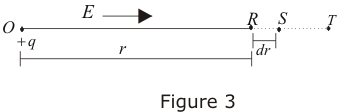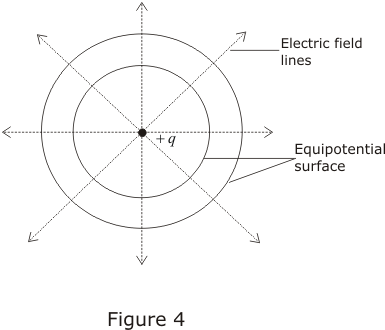Equipotential surface|Relation between electric fields and Electric potential

5. Relation between electric fiels and electric potential

• Consider the electric field E due to a point charge +q at point O in a radially outward direction shown below in the figure.• Suppose R and S are two points at a distance r and r+dr from point O where dr is vanishingly small distance and V is electric potential at point R.
• Now force on any test charge q' at point R in terms of electric field is
F=q'E
• Work done by the force in displacing test charge from R to S in field of charge q is
dW = F·dr = q'E·dr
and, change in potential energy is
dU = -dW = -q'E·dr
Change in electric potential would be
dV = dU/q
or dV = -E·dr                                             (11)
• From equation 11 electric field is
E=-(dV/dr)                                                    (12)
the quantity dV/dr is the rate of change of potential with the distance and is known as potential gradient. Negative sign in equation 12 indicates the decrease in electric potential in the direction of electric field.
• For cartesian coordinate system
E=Exi+Eyj+Ezk
and,
dr=dxi+dyj+dzk
from equation 11
dV=-E⋅dr
or, dV=-(Exdx+Eydy+Ezdz)          (13)
• Thus components of E are related to corresponding derivatives of V in the following manner
Ex=dV/dx                                          (14a)
Ey=dV/dy                                          (14b)
Ez=dV/dz                                          (14c)
In equation (14a) we see that V is differentiated with respect to coordinate x keeping other coordinates constant. Same is the case with equations (14b) and (14c) in case of y and z coordinates respectively.

6. Equipotential surfaces

• Surface over which the electric potential is same everywhere is called an equipotential surface.
• Equipotential surfaces are graphical way to represent potential distribution in an electric field.
• We can draw equipotential surfaces through a space having electric field.
• For a positive charge , electric field would be in radially outward direction and the equipotential surfaces would be concentric spheres with centers at the charge as shown below in the figure.• Since electric potential remains same everywhere on an equipotential surface from this it follows that PE of a charged body is same at all points on this surface.This shows that work done in moving a charged body between two points on an equipotential surface would be zero.
• At every point on equipotential surface electric field lines are perpandicular to the surface. This is because potential gradient along any direction parallel to the surface is zero i.e.,
E=-dV/dr=0
so component electric parallel to equipotential surface is zero.